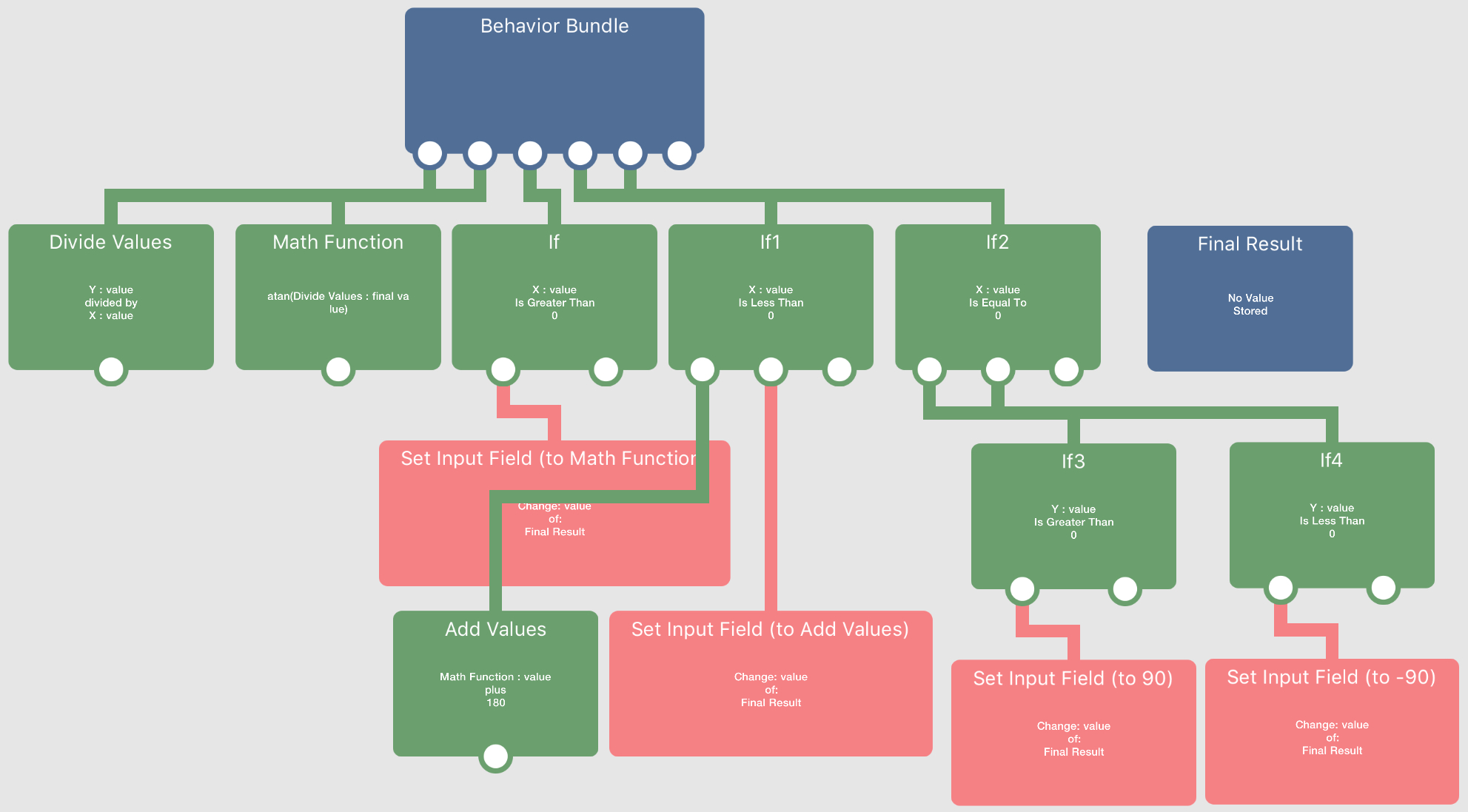Get Velocity -> Convert to a Direction

• I suck at math.

And mathematics, too.

How do I convert a Velocity into a direction?

• @Deeeds if you just want a formula, there's this one:

2Atan(x/(Sqrt(x^2+y^2)+y))

It's based on the tangent half angle identities, but I don't remember exactly how it works. It incorrectly returns 0 rather than +/-180 for y<=0 when x=0 (divide by zero), so you'd have to use ifs to account for that if that's a problem.

Here it is in behaviour form without a check for 180°:• @Jack8680 Wouldn’t it be easier to just use angle = arctan(y ÷ x) with conditionals?

• @Aidan-Oxley yes. I don't remember why I use such a complicated formula, it still has a conditional anyway.

• @Jack8680 This is amazing.

Floored. THANK YOU!!!

I want my MTV.

• @Aidan-Oxley This gives very funky results. I have tried adding 90 to the result, but this only works for an initial call. Live updating after are inverted causing the object to rotate in the opposite direction to the velocity direction changes.

• @Deeeds My formula needs conditionals, Jack’s does not. I think conditionals are more efficient in power requirement than square roots. The conditionals you’d need are If x > 0, x < 0, x = 0 (and for the x = 0 case you’d need 2 more for if y is negative or positive). Depending on how your project works, the x=0 case could be extremely rare and possibly could be ignored depending on what you’re doing with the angle. I’ll send a screenshot showing exactly what I mean.

•I have no idea which is more efficient in processing power, but this way is definitely less straight forward than just using a formula. I’d say just stick with what you have now, it’s probably not much different.

• @Aidan-Oxley Imagine throwing a ball into the air, at an angle, and ball being smart enough to look in the direction its travelling, as that changes through the arc of its flight. Then continue doing the same when it bounces, hits a wall and reverses, or does anything a ball might do.

That's what I'm trying to do.

But it doesn't seem to work with any of these. Sometimes it reverses (starts fine then looks the other way as the direction changes) sometimes it's doing stuff I can't explain, other times it's off by 90 degrees at start, but correcting this has weird effects, and doesn't last.

• @Deeeds I haven’t tried using/testing Jacks formula, but I’m quite sure that my one (with the heap of conditionals) will work. The output might be a bit strange, but that’s because there’s no point in converting for example the angle of -30° into 330°, but the two angles are the same. If it’s still being strange with both my formula and Jack’s, I’ll make an example project of launching a ball as you said with an arrow pointing in it’s direction of travel.